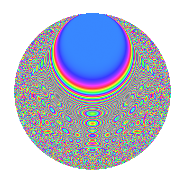# Properties

 Label 8042.2.aLevel 8042 Weight 2 Character orbit a Rep. character $$\chi_{8042}(1,\cdot)$$ Character field $$\Q$$ Dimension 336 Newform subspaces 4 Sturm bound 2011 Trace bound 1

# Related objects

## Defining parameters

 Level: $$N$$ = $$8042 = 2 \cdot 4021$$ Weight: $$k$$ = $$2$$ Character orbit: $$[\chi]$$ = 8042.a (trivial) Character field: $$\Q$$ Newform subspaces: $$4$$ Sturm bound: $$2011$$ Trace bound: $$1$$

## Dimensions

The following table gives the dimensions of various subspaces of $$M_{2}(\Gamma_0(8042))$$.

Total New Old
Modular forms 1007 336 671
Cusp forms 1004 336 668
Eisenstein series 3 0 3

The following table gives the dimensions of the cuspidal new subspaces with specified eigenvalues for the Atkin-Lehner operators and the Fricke involution.

$$2$$$$4021$$FrickeDim.
$$+$$$$+$$$$+$$$$82$$
$$+$$$$-$$$$-$$$$86$$
$$-$$$$+$$$$-$$$$101$$
$$-$$$$-$$$$+$$$$67$$
Plus space$$+$$$$149$$
Minus space$$-$$$$187$$

## Trace form

 $$336q - 2q^{3} + 336q^{4} - 2q^{5} + 334q^{9} + O(q^{10})$$ $$336q - 2q^{3} + 336q^{4} - 2q^{5} + 334q^{9} - 12q^{11} - 2q^{12} + 10q^{13} + 4q^{14} + 4q^{15} + 336q^{16} + 8q^{17} + 8q^{18} + 8q^{19} - 2q^{20} + 20q^{21} - 6q^{22} + 4q^{23} + 330q^{25} + 4q^{26} + 4q^{27} - 12q^{30} - 12q^{31} + 12q^{33} - 8q^{34} - 4q^{35} + 334q^{36} + 2q^{37} - 2q^{38} + 16q^{39} - 4q^{41} - 8q^{42} - 12q^{43} - 12q^{44} - 6q^{45} + 4q^{46} + 24q^{47} - 2q^{48} + 340q^{49} + 4q^{51} + 10q^{52} + 14q^{53} - 4q^{55} + 4q^{56} + 28q^{57} - 2q^{58} - 46q^{59} + 4q^{60} + 30q^{61} + 20q^{62} + 48q^{63} + 336q^{64} + 16q^{65} + 22q^{67} + 8q^{68} + 20q^{69} - 4q^{70} + 8q^{71} + 8q^{72} + 24q^{74} + 18q^{75} + 8q^{76} - 16q^{77} + 4q^{78} - 8q^{79} - 2q^{80} + 320q^{81} + 12q^{82} + 2q^{83} + 20q^{84} - 20q^{85} + 30q^{86} - 12q^{87} - 6q^{88} + 16q^{89} + 4q^{90} + 36q^{91} + 4q^{92} + 20q^{93} - 12q^{94} - 20q^{95} - 12q^{97} + 40q^{98} - 132q^{99} + O(q^{100})$$

## Decomposition of $$S_{2}^{\mathrm{new}}(\Gamma_0(8042))$$ into newform subspaces

Label Dim. $$A$$ Field CM Traces A-L signs $q$-expansion
$$a_2$$ $$a_3$$ $$a_5$$ $$a_7$$ 2 4021
8042.2.a.a $$67$$ $$64.216$$ None $$67$$ $$-11$$ $$-20$$ $$-40$$ $$-$$ $$-$$
8042.2.a.b $$82$$ $$64.216$$ None $$-82$$ $$-13$$ $$3$$ $$-37$$ $$+$$ $$+$$
8042.2.a.c $$86$$ $$64.216$$ None $$-86$$ $$12$$ $$-4$$ $$35$$ $$+$$ $$-$$
8042.2.a.d $$101$$ $$64.216$$ None $$101$$ $$10$$ $$19$$ $$42$$ $$-$$ $$+$$

## Decomposition of $$S_{2}^{\mathrm{old}}(\Gamma_0(8042))$$ into lower level spaces

$$S_{2}^{\mathrm{old}}(\Gamma_0(8042)) \cong$$ $$S_{2}^{\mathrm{new}}(\Gamma_0(4021))$$$$^{\oplus 2}$$

## Hecke Characteristic Polynomials

There are no characteristic polynomials of Hecke operators in the database